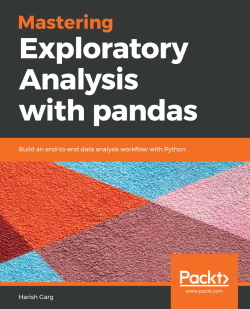# Mastering Exploratory Analysis with pandas

By Harish Garg
• \$5/mo for 5 months Subscribe Access now
• \$24.99 Print + eBook
• \$17.99 eBook

• Constantly updated with 100+ new titles each month
• Breadth and depth in over 1,000+ technologies

The pandas is a Python library that lets you manipulate, transform, and analyze data. It is a popular framework for exploratory data visualization and analyzing datasets and data pipelines based on their properties.

This book will be your practical guide to exploring datasets using pandas. You will start by setting up Python, pandas, and Jupyter Notebooks. You will learn how to use Jupyter Notebooks to run Python code. We then show you how to get data into pandas and do some exploratory analysis, before learning how to manipulate and reshape data using pandas methods. You will also learn how to deal with missing data from your datasets, how to draw charts and plots using pandas and Matplotlib, and how to create some effective visualizations for your audience. Finally, you will wrapup your newly gained pandas knowledge by learning how to import data out of pandas into some popular file formats.

By the end of this book, you will have a better understanding of exploratory analysis and how to build exploratory data pipelines with Python.

Publication date:
September 2018
Publisher
Packt
Pages
140
ISBN
9781789619638

# Working with Different Kinds of Datasets

In this chapter, we will learn how to work with different kinds of dataset formats in pandas. We'll learn how to use the advanced options provided by pandas' imported CSV files. We will also look at how to work with Excel files in pandas, and how to use advanced options of the read_excel method. We'll explore some of the other pandas methods for working with popular data formats, such as HTML, JSON, PICKLE files, SQL, and so on.

In this section, we will be working with CSV datasets and learn how to import CSV datasets as well as advanced options for pandas: the read_csv method.

# Importing modules

First, we will start by importing the pandas module with the following command:

`import pandas as pd`

To read the CSV files, we use the read_csv method, as follows:

`df = pd.read_csv('IMDB.csv', encoding = "ISO-8859-1")df.head()`

In order to perform a basic import, pass the filename of the dataset to read_csv, and assign the resulting DataFrame to a variable. In the following screenshot, we can see that pandas has turned the dataset into a tabular format: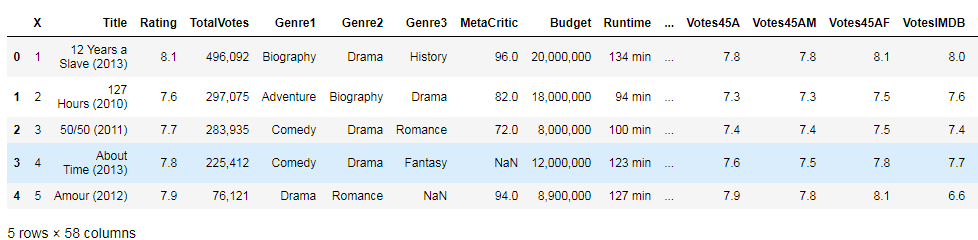In Python, pandas has a lot of advanced options for the read_csv method, which is where you can control how the data is read from a CSV file.

# Manipulating columns, index locations, and names

By default, read_csv considers the entries in the first row of the CSV file as column names. We can turn this off by setting header to None, as shown in the following code block:

`df = pd.read_csv('IMDB.csv', encoding = "ISO-8859-1", header=None)df.head()`

The output is as follows:# Specifying a different row as a header

You can also set the column name from a different row—instead of the default first row—by passing the row number to the header option, as follows:

`df = pd.read_csv('IMDB.csv', encoding = "ISO-8859-1", header=2)df.head()`

The output is as follows: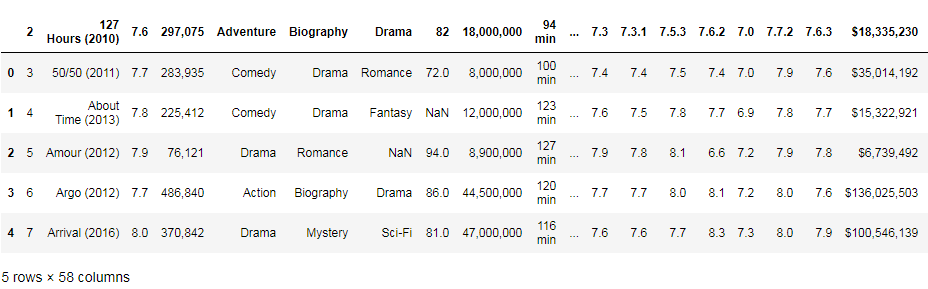# Specifying a column as an index

By default, read_csv assigns a default numeric index starting with zero while reading the data. However, you can change this behavior by passing the column name to the index column option. pandas will then set the index to this column, as shown in the following code:

`df = pd.read_csv('IMDB.csv', encoding = "ISO-8859-1", index_col='Title')df.head()`

Here, we passed a movie title as the index name. Now the index name is Title, instead of a default numeric index, as shown in the following screenshot: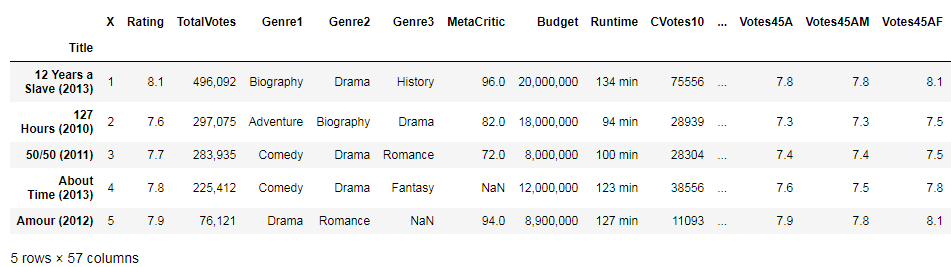# Choosing a subset of columns to be read

We can also choose to read a specific subset of columns in the CSV file. For this, we pass the column names as a list to use the columns option, as follows:

`df = pd.read_csv('IMDB.csv', encoding = "ISO-8859-1", usecols=['Title', 'Genre1'])df.head()`

Output of the preceding code snippet is as follows:# Handling missing and NA data

Next, we will see how to handle missing data by reading a CSV file. By default, read_csv considers the following values missing and marks them as NaN: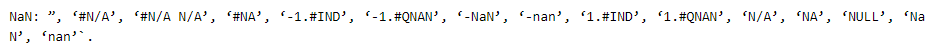However, you can add to this list. To do so, simply pass the list of values you want to be considered as NaN, as shown in the following code:

`df = pd.read_csv('IMDB.csv', encoding = "ISO-8859-1", na_values=[''])`

# Choosing whether to skip over blank rows

Sometimes whole rows have no values; hence, we can choose what to do with these rows while reading data. By default, read_csv ignores blank rows, but we can turn this off by setting skip_blank_lines to False, as follows:

`df = pd.read_csv('IMDB.csv', encoding = "ISO-8859-1", skip_blank_lines=False)`

# Data parsing options

We can choose which rows are skipped by reading a CSV file. We can pass the row numbers as a list to the skiprows option. The first row has the index zero, as follows:

`df = pd.read_csv('IMDB.csv', encoding = "ISO-8859-1", skiprows = [1,3,7])df.head()`

The output is as follows: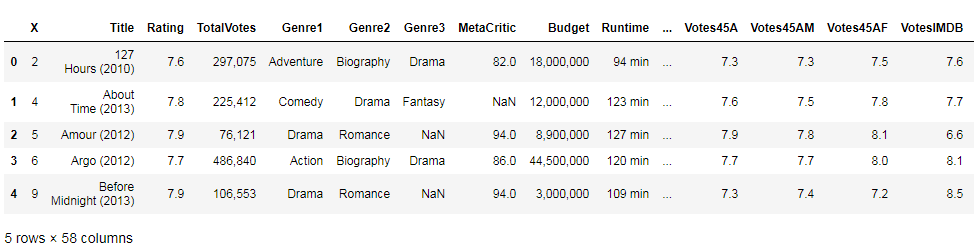# Skipping rows from the footer or end of the file

To skip rows from the footer or the end of a file, use the skipfooter option and pass a number specifying the number of rows to skip. In the following code, we have passed 2. As we can see, there is a difference between the previous DataFrame we created and the DataFrame we created after skipping the last two rows:

`df.tail(2)df = pd.read_csv('IMDB.csv', encoding = "ISO-8859-1", skipfooter=2, engine='python')df.tail(2)`

The following screenshot shows the output: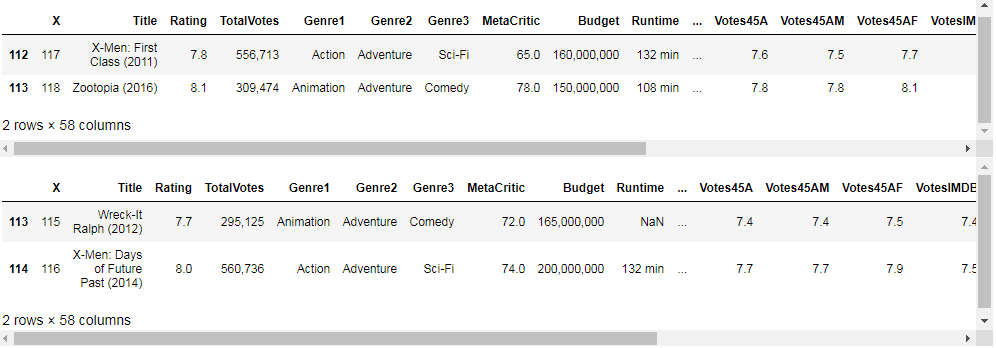# Reading the subset of a file or a certain number of rows

Sometimes a data file is too big, and we just want to have a peek at the first few rows. We can do that by passing the number of rows to import to the nrows option, as shown in the following code. Here, we passed 100 to nrows, which then read only the first hundred rows from the dataset:

`df = pd.read_csv('IMDB.csv', encoding = "ISO-8859-1", nrows=100)df.shape`

# Reading data from Excel files

In this section, we will learn how to work with Excel data using pandas and use pandas' read_excel method for reading data from Excel files. We will read and explore a real Excel dataset and explore some of the advanced options available for parsing Excel data.

pandas internally uses the Excel rd Python library to extract data from Excel files. We can install it by executing conda install xlrd.

Firstly, make sure the command-line program is running in admin mode ahead of installation, as shown in the following screenshot: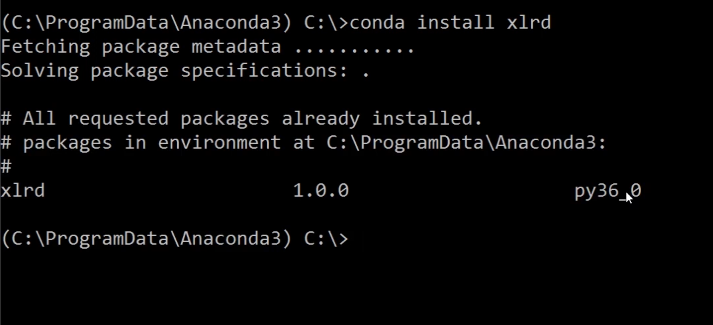The following screenshot shows the Excel dataset that we will be reading and exploring with pandas: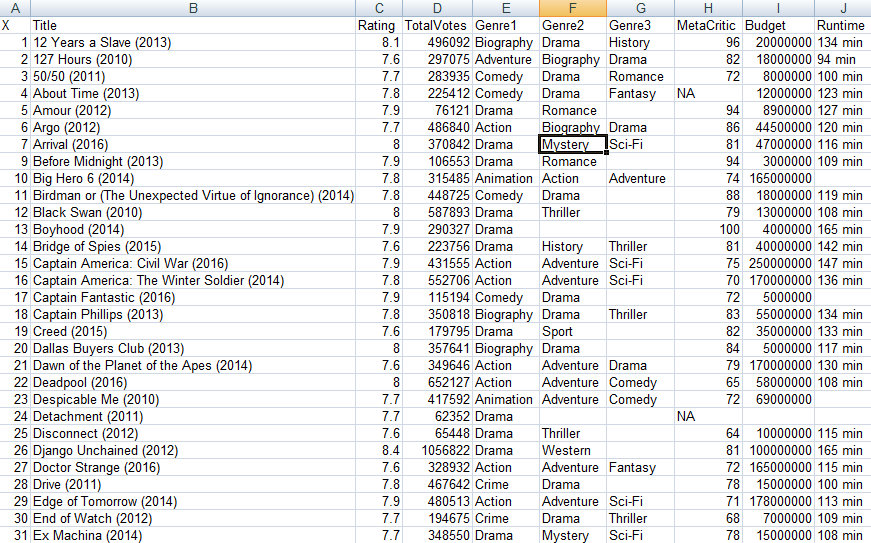Previous screenshot is collection of Movie rating which can be found at: https://github.com/saipranava/IMDB.

We are using pandas' read_excel method to read this data. In its simplest format, we are just passing the filename of the Excel dataset we want to the read_excel method. pandas converts the data from the Excel file into a pandas DataFrame . The pandas internally uses the Excel rd library for this. Here, pandas has read the data and created a tabular data object in the memory, which we can access, explore, and manipulate in our code, as shown in the following code:

`df = pd.read_excel('IMDB.xlsx')df.head()`

The output for the previous code block is as follows: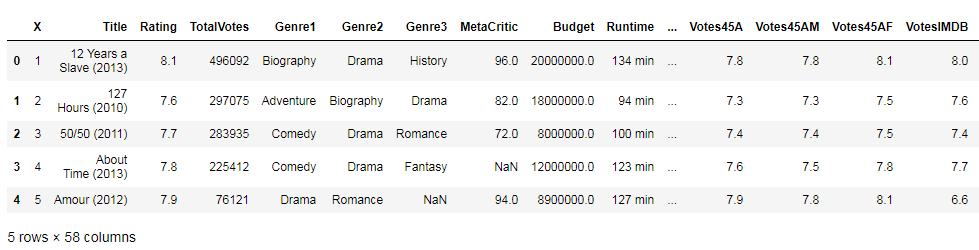pandas has a lot of advanced options, which we can use to control how data should be read, as shown in the following screenshot: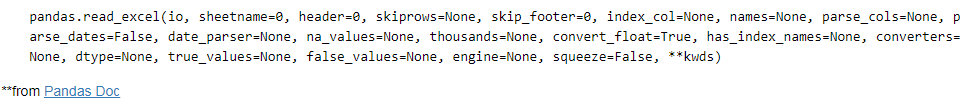# Specifying which sheet should be read

To specify which sheet should be read, pass the value to the sheetname option. As you can see in the following screenshot, we are simply passing 0, which is the index value of the first sheet in the Excel sheet. This is quite handy, especially when we don't know the exact sheet name:

`df = pd.read_excel('IMDB.xlsx', sheetname=0)df.head()`

The output is as follows: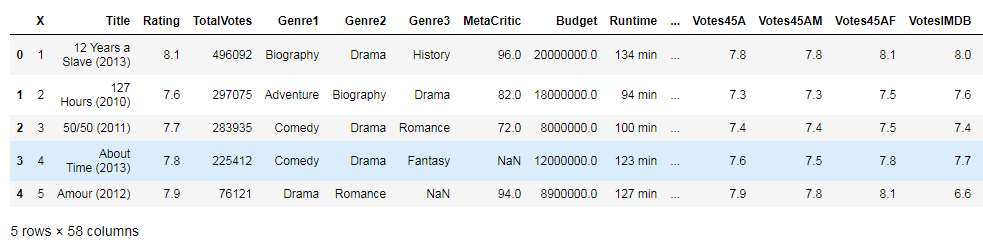# Reading data from multiple sheets

Excel dataset files come with data and multiple sheets. In fact, this is one of the main reasons a lot of users prefer Excel over CSV. Luckily, pandas supports the reading of data from multiple sheets.

# Finding out sheet names

To find out the name of a sheet, pass the Excel file to the ExcelFile class and call the sheet_names property on the resulting object. The class prints the sheet names from the Excel file as a list. If we want to read data from a sheet named data-movies, it will look like the following code snippet:

`xls_file = pd.ExcelFile('IMDB.xlsx')xls_file.sheet_names`

Next, we call the parse method on the Excel file object we created earlier, and pass in the sheet names we want to read. We then assign the result to two separate DataFrame objects, as follows:

`df1 = xls_file.parse('movies')df2 = xls_file.parse('by genre')df1.head()`

We now have our data from two sheets in two separate DataFrames, as shown in the following screenshot: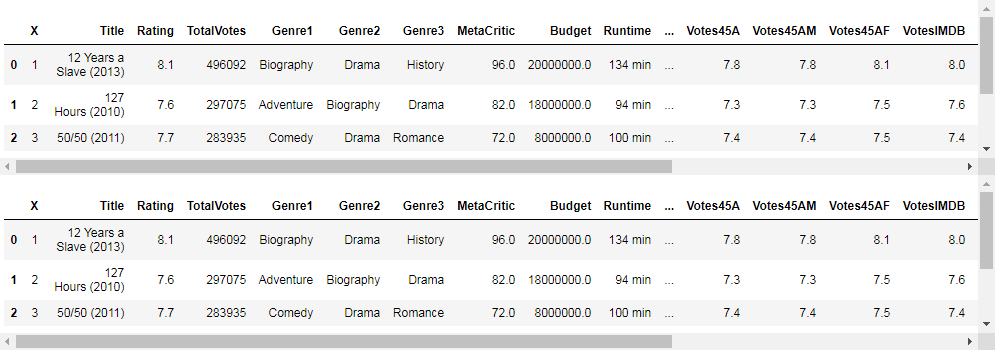# Choosing header or column labels

pandas will, by default, set the column names or header to the values from the first non-blank row in the Excel file. However, we can change this behavior. In the following screenshot, we are passing the value 3 to the header option, which tells the read_excel method to set the header names from index row 3:

`df = pd.read_excel('IMDB.xlsx', sheetname=1, header=3)df.head()`

The output of the preceding code is as follows: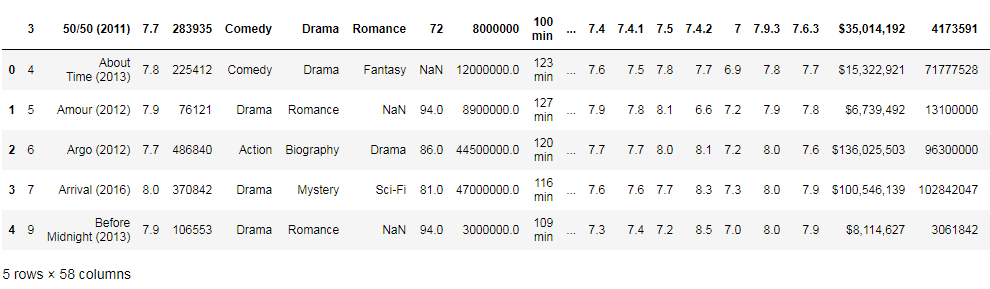We can also tell read_excel to ignore the header and treat all rows as records. This is handy whenever Excel has no header row. To achieve this, we set header to None, as shown in the following code:

`df = pd.read_excel('IMDB.xlsx', sheetname=1, header=None)df.head()`

The output is as follows: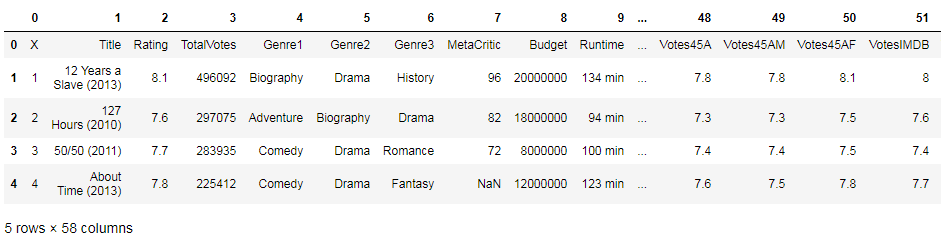# Skipping rows at the beginning

To skip rows at the beginning of a file, we simply set skiprows to the number of rows we want to skip, as shown in the following code:

`df = pd.read_excel('IMDB.xlsx', sheetname=1, skiprows=7)`

# Skipping rows at the end

For this, we use the skip_footer option, as follows:

`df = pd.read_excel('IMDB.xlsx', sheetname=1, ski_footer=10)`

# Choosing columns

We can also choose to read only a subset of columns. This is done by setting the parse_cols option to a numeric value, which will lead to columns being read from 0 to whichever index we set the parse columns value to. We set parse_cols=2 in this instance, which reads the first three columns in the Excel file, as shown in the following code snippet:

`df = pd.read_excel('IMDB.xlsx', sheetname= 0, parse_cols=2)df.head()`

The following is the output:# Column names

We can choose to give different names to columns instead of the default names given in the header row. To do this, we pass the list of column names to the names parameter, as follows:

`df = pd.read_excel('IMDB.xlsx', sheetname=0, parse_cols = 2, names=['X','Title',   'Rating'], )df.head()`

In the following screenshot, we have set column names to the names we passed while reading: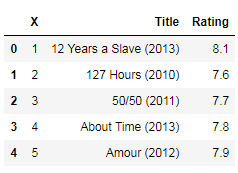# Setting an index while reading data

By default, read_excel labels zeros with the numeric index, starting with 0. We can set our index or row labels to a higher value or to our choosing. To do this, we pass a column name from our dataset to the index_col option. In the following code, we are setting our index to the Title column:

`df = pd.read_excel('IMDB.xlsx', sheetname=0, index_col='Title')df.head()`

The output is as follows: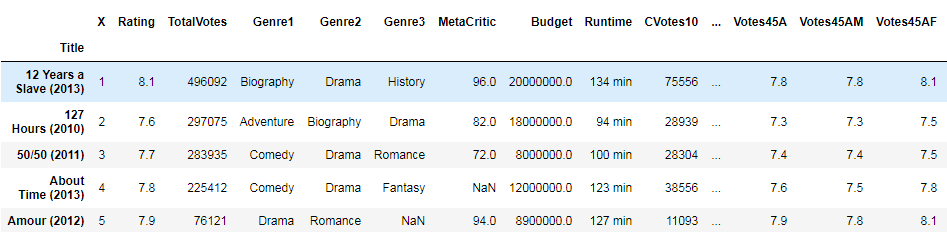# Handling missing data while reading

The read_excel method has a list of values that it will consider as missing and will then set the values to NaN. We can add this when passing a list of values by using the na_values parameter, as shown in the following code:

`df = pd.read_excel('IMDB.xlsx', sheetname= 0, na_values=[' '])`

# Reading data from other popular formats

In this section, we will explore pandas' features for reading and working with various popular data formats. We will also learn how to read data from the JSON format, HTML files, and the PICKLE dataset and how to read data from an SQL-based database.

JSON is a minimal readable format for structuring data. It is used primarily to transmit data between a server and web application as an alternative to XML, as shown in the following screenshot: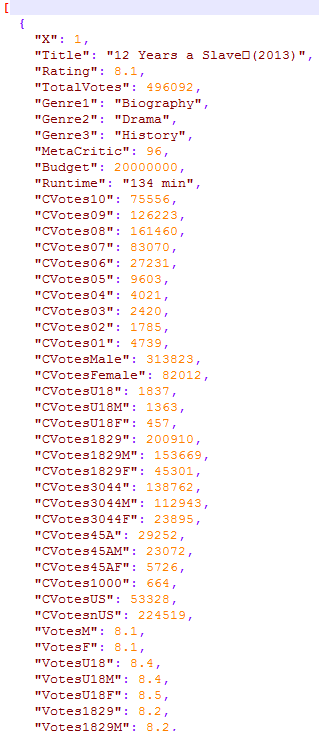# Reading JSON data into pandas

To read JSON data, pandas provides a method called read_json, where we pass the filename and location of the JSON data file we want to read. The file location can be local, or even on the internet with a valid URL scheme. We assign the resulting DataFrame to the variable DF.

The read_json method reads the JSON data and converts it into a pandas DataFrame object, a tabular data format, as shown in the following code. The JSON data, which is now easily accessible in a DataFrame format, can be manipulated and explored with greater ease:

`movies_json = pd.read_json('IMDB.json')movies_json.head()`

The previous code block will produce the following output: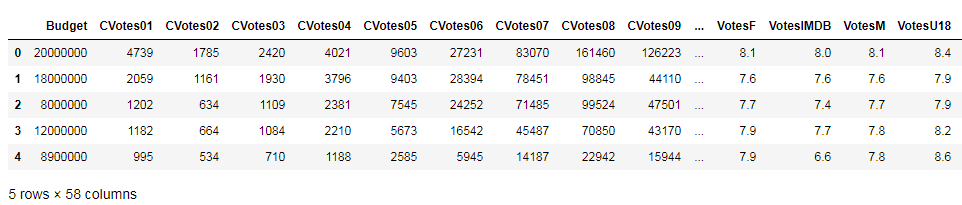pandas uses the lxml Python module internally to read HTML data. You can install it from the command- line program by executing conda install lxml, as shown in the following screenshot: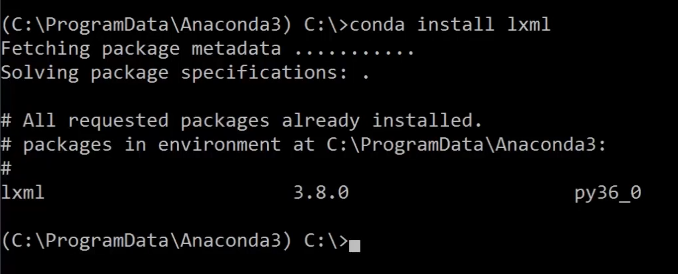We can also import HTML data from local files, or even directly from the internet, as well: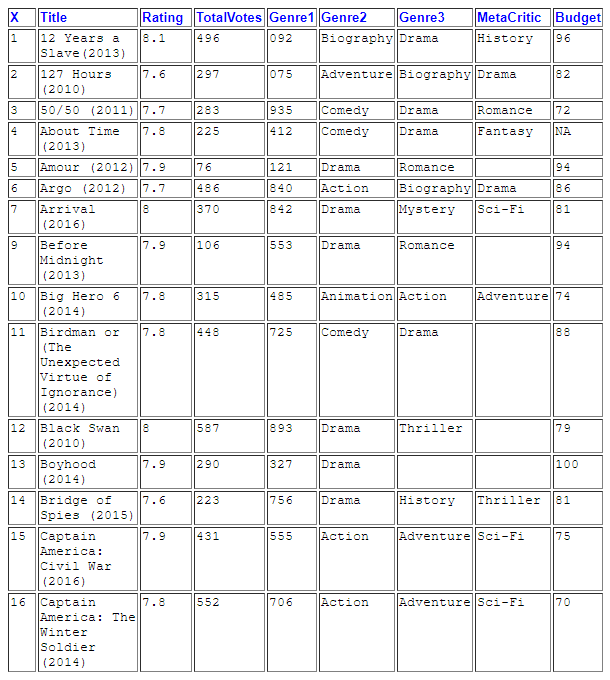Here, we pass in the location of the HTML file, or the URL, to the read_html method. read_html extracts the tabular data from HTML, and then converts it into a pandas DataFrame . In the following code, we have the data we extracted from the HTML file in a tabular format:

`pd.read_html('IMDB.html')`

The output is as follows: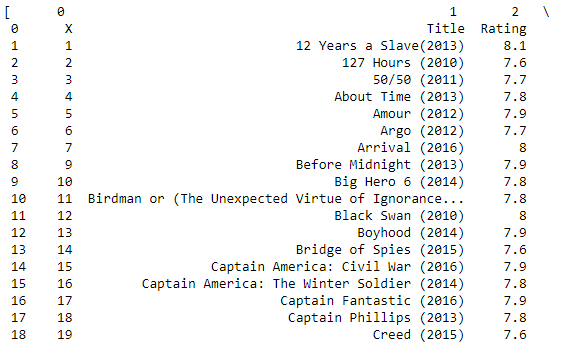Pickling is a way to convert a Python object of any type, including a list, dictionary, and so on, into a character string. The idea is that this character string contains all the information necessary for reconstructing the object in another Python script.

We use read_pickle method to read our PICKLE file, as shown in the following code. As with other data formats, pandas creates a DataFrame from the read data:

`df = pd.read_pickle('IMDB.p')df.head()`

The output is as follows: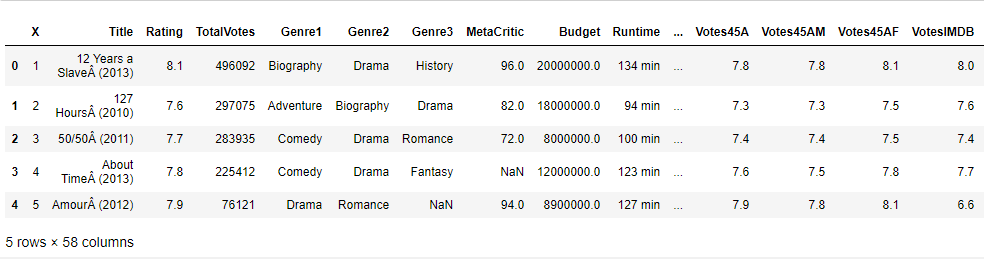Here we will be reading SQL data from the popular database browser that is SQLite which can be installed by executing the following command:

`conda install sqlite`

Then we will import the SQLite Python module as follows:

`import sqlite3`

Then, create a connection to the SQLite DB you want to read data from, as follows:

`conn = sqlite3.connect("IMDB.sqlite")df = pd.read_sql_query("SELECT * FROM IMDB;", conn)df.head()`

Next, pass the SQL query you want the data from to pandas with the read_sql_query method. The method reads the data and creates a DataFrame object, as shown in the following screenshot: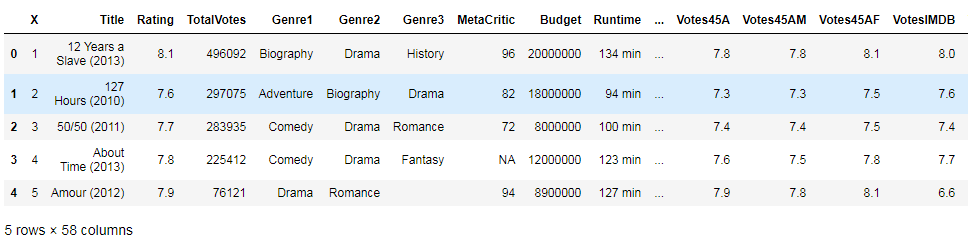An SQLite database was used for this demo, but you can read data from other databases too. To do so, just call the appropriate DB Python module.

# Reading data from the clipboard

To read data from the clipboard, first copy some data. In the following example, we have copied a table from a the movies dataset: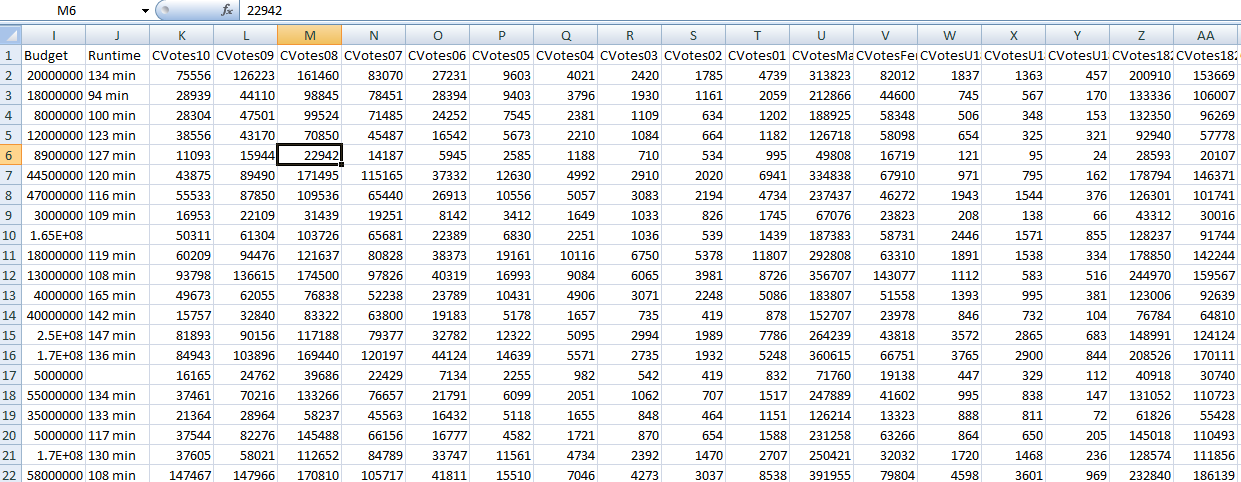Next, use pandas' read_clipboard method to read the data and create a DataFrame , as follows:

`df = pd.read_clipboard()df.head()`

The data copied from the web page is now in memory as a DataFrame , as shown in the following screenshot. This method is quite handy when it comes to bringing data in quickly to pandas:# Summary

In this chapter, we learned how to work with different kinds of dataset formats in pandas. We learned how to use the advanced options provided by pandas while importing CSV files. We also saw how to work with Excel datasets, and we explored the methods available for working with various data formats such as HTML, JSON, PICKLE files, SQL, and so on.

In the next chapter, we will learn how to use pandas techniques in advanced data selection.